# Maharashtra Board Class 12 Chemistry Solutions Chapter 9 Coordination Compounds

Balbharti Maharashtra State Board 12th Chemistry Textbook Solutions Chapter 9 Coordination Compounds Textbook Exercise Questions and Answers.

## Maharashtra State Board Class 12 Chemistry Solutions Chapter 9 Coordination Compounds

1. Choose the most correct option.

Question i.
The oxidation state of cobalt ion in the complex [Co(NH3)5Br]SO4 is ……………………….
a. + 2
b. + 3
c. + 1
d. + 4
(b) + 3Question ii.
IUPAC name of the complex [Pt(en)2(SCN)2]2+ is ………………………
a. bis (ethylenediamine dithiocyanatoplatinum (IV) ion
b. bis (ethylenediamine) dithiocyantoplatinate (IV) ion
c. dicyanatobis (ethylenediamine) platinate IV ion
d. bis (ethylenediammine)dithiocynato platinate (IV) ion
(a) bis(ethylenediamine dithiocyanatoplatinum (IV) ion

Question iii.
Formula for the compound sodium hexacynoferrate (III) is
a. [NaFe(CN)6]
b. Na2[Fe(CN)6]
c. Na[Fe(CN)6]
d. Na3[Fe(CN)6]
(d) Na3[Fe(CN)6]

Question iv.
Which of the following complexes exist as cis and trans isomers?
1. [Cr(NH2)2Cl4]
2. [Co(NH3)5Br]2⊕
3. [PtCl2Br2]2⊕ (square planar)
4. [FeCl2(NCS)2]2⊕ (tetrahedral)
a. 1 and 3
b. 2 and 3
c. 1 and 3
d. 4 only
(a) 1 and 3

Question v.
Which of the following complexes are chiral?
1. [Co(en)2Cl2]
2. [Pt(en)Cl2]
3. [Cr(C2O4)3]3⊕
4. [Co(NH3)4CI2]
a. 1 and 3
b. 2 and 3
c. 1 and 4
d. 2 and 4
(a) 1 and 3

Question vi.
On the basis of CFT predict the number of unpaired electrons in [CrF6]3.
a. 1
b. 2
c. 3
d. 4
(c) 3

Question vii.
When an excess of AgNO3 is added to the complex one mole of AgCl is precipitated. The formula of the complex is ……………..
a. [CoCl2(NH3)4]Cl
b. [CoCl(NH3)4] Cl2
c. [CoCl3(NH3)3]
d. [Co(NH3)4]Cl3
(a) [COCI3(NH3)4]CI

Question viii.
The sum of coordination number and oxidation number of M in [M(en)2C2O4]Cl is
a. 6
b. 7
c. 9
d. 8
(c) 92. Answer the following in one or two sentences.

Question i.
Write the formula for tetraammineplatinum (II) chloride.
Formula of tetraamineplatinum(II) chloride : [Pt(NH3)4]CI2

Table 9.1 : IUPAC names of anionic and neutral ligands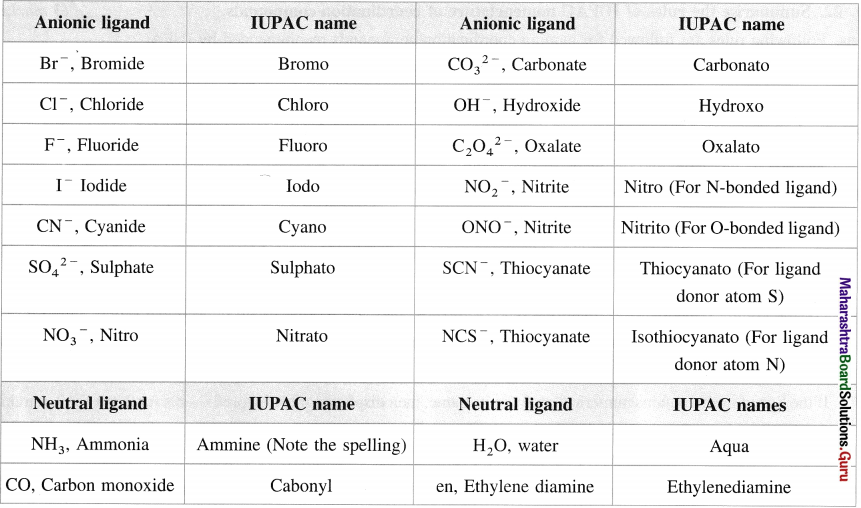Table 9.2: IUPAC names of anionic complexes

 Metal Name A1 Cr Cu Co Au(Gold) Fe Pb Mn Mo Ni Zn Ag Sn Aluminate Chromate Cuprate Cobaltate Aurate Ferrate Plumbate Manganate Molybdate Nickelate Zincate Argentate Stannate

Table 9.3 : IUPAC names of some complexes

 Complex IUPAC name (i) Anionic complexes : (a) [Ni(CN)J2- (b) [Co(C204)3]3- (c) [Fe(CN)6]4- Tetracyanonickelate(II) ion Trioxalatocobaltate(III) ion Hexacyanoferrate(II) ion (ii) Compounds containing complex anions and metal cations : (a) Na3[Co(N02)6] (b) K3[A1(C204)3] (c) Na3[AIF6] Sodium hexanitrocobaltate(III) Potassium trioxalatoaluminate(III) Sodium hexafluoroaluminate(III) (iii) Cationic complexes : (a) [Cu(NH3)4]2+ (b) [Fe(H20)5(NCS)]2+ (c) [Pt(en)2(SCN)2]2+ Tetraamminecopper(II) ion Pentaaquai sothiocyanatoiron(III) ionBis(ethylenediamine)dithiocyanatoplatinum(IV) (iv) Compounds containing complex cation and anion : (a) [PtBr2(NH3)4]Br2 (b) [Co(NH3)5C03]CI (c) [Co(H20)(NH3)5]I3 Tetraamminedibromoplatinum(IV) bromide, Pentaamminecarbonatocobalt(III) chloride, Pentaammineaquacobalt(III) iodide (v) Neutral complexes : (a) Co(N02)3(NH3)3 (b) Fe(CO)5 (c) Rh(NH3) 3(SCN) 3 Triamminetrinitrocobalt(III) Pentacarbonyliron(0) Triamminetrithiocyanatorhodium(III)Question ii.
Predict whether the [Cr(en)2(H2O)2]3+ complex is chiral. Write structure of its enantiomer.
(i) Complex is chiral.
(ii) The following are its enantiomers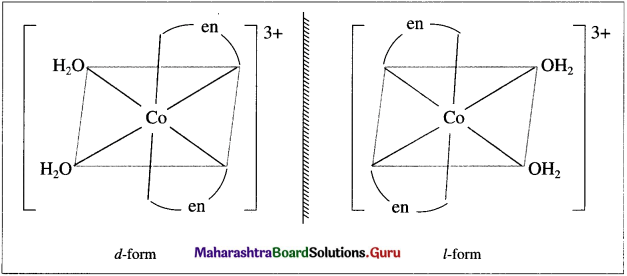Question iv.
Name the Lewis acids and bases in the complex [PtCl2(NH3)2].
Lewis acid : Pt2+
Lewis bases : Cl and NF3

Question v.
What is the shape of a complex in which the coordination number of central metal ion is 4?
A complex with the coordination number of central metal ion equal to 4 may be tetrahedral or square planar.

Question vi.
Is the complex [CoF6] cationic or anionic if the oxidation state of cobalt ion is +3?
In the complex, Co carries + 3 charge while 6F carry – 6 charge. Hence the net charge on the complex is – 3.
Therefore it is an anionic complex.

Question vii.
Consider the complexes [Cu(NH3)4][PtCl4] and [Pt(NH3)4] [CuCl4]. What type of isomerism these two complexes exhibit?
Since in these two given complexes, there is an exchange of ligands between cationic and anionic constituents, they exhibit coordination isomerism.

Question viii.
Mention two applications of coordination compounds.
(1) In biology : Several biologically important natural compounds are metal complexes which play an important role in number of processes occurring in plants and animals.

For example, chlorophyll in plants is a complex of Mg2+ ions, haemoglobin in blood is a complex of iron, vitamin B12 is a complex of cobalt.

(2) In medicine : The complexes are used on a large scale in medicine. Many medicines in the complex form are more stable, more effective and can be assimilated easily.

For example, platinum complex [Pt(NH3)2CI2] known as cisplatin is effectively used in cancer treatment. EDTA is used to treat poisoning by heavy metals like lead.

(3) To estimate hardness of water :

• The hardness of water is due to the presence Mg2+ and Ca2+ ion in water.
• The strong field ligand EDTA forms stable complexes with Mg2+ and Ca2+. Hence these ions can be removed by adding EDTA to hard water.

Similarly these ions can be selectively estimated due to the difference in their stability constants.

(4) Electroplating : This involves deposition of a metal on the other metal. For smooth plating, it is necessary to supply continuously the metal ions in small amounts.
For this purpose, a solution of a coordination compound is used which dissociates to a very less extent. For example, for uniform and thin plating of silver and gold, the complexes K[Ag(CN)2] and K[Au(CN)2] are used.

Question i.
What are bidentate ligands? Give one example.
Bidentate ligand : This ligand has two donor atoms in the molecule or ion. For example, ethylenediamine, H2N – (CH2)2 – NH2.

Question ii.
What is the coordination number and oxidation state of metal ion in the complex [Pt(NH3)Cl5]2?
Coordination number = 6
Oxidation state of Pt = +4.Question iii.
What is the difference between a double salt and a complex? Give an example.

 Double salt Coordination compound (complex) (1) Double salts exist only in the solid state and dissociate into their constituent ions in the aqueous solutions. (1) Coordination compounds exist in the solid-state as well as in the aqueous or non-aqueous solutions. (2) Double salts lose their identity in the solution. (2) They do not lose their identity completely. (3) The properties of double salts are same as those of their constituents. (3) The properties of coordination compounds are different from their constituents. (4) Metal ions in the double salts show their normal valence. (4) Metal ions in the coordination compounds show two valences namely primary valence and second­ary valence satisfied by anions or neutral molecules called ligands. (5) For example in K2SO4. K2SO4. A12(SO4)3. 24H2O. The ions K+, Al3 + and SO4 show their properties. (5) In K4[Fe(CN)6], ions K+ and [Fe(CN)6]4‘~ ions show their properties.

Question iv.
Classify the following complexes as homoleptic and heteroleptic
[Cu(NH3)4]SO4, [Cu(en)2(H2O)Cl]3⊕, [Fe(H2O)5(NCS)]2⊕, tetraammine zinc (II) nitrate.
Homoleptic complex :
(a) [Cu(NH3)4]SO4
(d) Tetraaminezinc (II) nitrate : [Zn(NH3)4](NO3)2

Heteroleptic Complex :
(b) [Cu(en)2(H2O)CI]2+
(c) [Fe(H2O)5(NCS)]2+

Question v.
Write formulae of the following complexes
a. Potassium ammine-tri chloroplatinate (II)
b. Dicyanoaurate (I) ion
(a) Potassium amminetrichloroplatinate(II) K[Pt(NH3)CI3]
(b) Dicyanoaurate (I) ion [AU(CN)2]

Question vi.
What are ionization isomers? Give an example.
Ionisation isomers : The coordination compounds having same molecular composition but differ in the compositions of coordination (or inner) sphere and outer sphere and produce different ions on ionisation in the solution are called ionisation isomers. For example, Pentaamminesulphatocobalt (III) bromide [Co(NH3)5SO4] Br, Pentaamminebromocobalt(III) sulphate [Co(NH3)5Br] SO4.

Question vii.
What are the high-spin and low-spin complexes?
(1) High spin complex (HS) :

• The complex which has greater iwmher of unpaired electrons and hence a higher value of resultant spin and magnetic moment is called high spin (or spin free) or IlS complex.
• It is formed with weak field ligands and the complexes have lower values for crystal field splitting energy (CFSE). Δ0
• The paramagnetism of HS complex is larger.

(2) Low spin complex (LS) :

• The complex which has the Icasi number of unpaired electrons or all electrons paired and hence the lowest
(or no) resultant spin or magnetic moment is called low spin (or spin paired) or LS complex.
• It is formed with strong tickl ligands and the complexes have higher values of crystal field splitting energy (Δ0).
• Low spin complex is diamagnetic or has low paramagnetism.

Table 9.5 : d-orbitai diagrams fir high spin and low spin complexes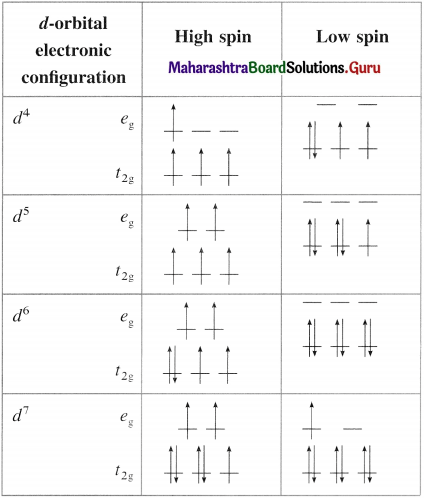(Only the electronic configurations c4 to d1 render the high spin and low spin complexes)Question viii.
[CoCl4]2⊕ is a tetrahedral complex. Draw its box orbital diagram. State which orbitals participate in hybridization.
27Co [Ar] 3d74s2
Oxidation state of Co = +2 Co2+ [Ar] 3d7 4s°
Since CI is a weak ligand, there is no pairing of electrons. Since C.N. is 4, there is sp3 hybridisation.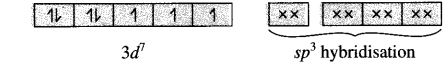Question ix.
What are strong field and weak field ligands? Give one example of each.
The ligands are then classified as (a) strong field and (b) weak field ligands. Strong field ligands are those in which donor atoms are C,N or P. Thus CN, NC, CO, HN3, EDTA, en (ethylenediammine) are considered to be strong ligands. They cause larger splitting of d orbitals and pairing of electrons is favoured. These ligands tend to form low spin complexes. Weak field ligands are those in which donor atoms are halogens, oxygen or sulphur.

For example, F, CI, Br, I, SCN, C2O42-. In case of these ligands the A0 parameter is smaller compared to the energy required for the pairing of electrons, which is called as electron pairing energy. The ligands then can be arranged in order of their increasing field strength as
I < Br < CI < S2- < F < OH < C2O42- < H2O < NCS < EDTA < NH3 < en < CN < CO.

Question x.
With the help of a crystal field energy-level diagram explain why the complex [Cr(en)3]3⊕ is coloured?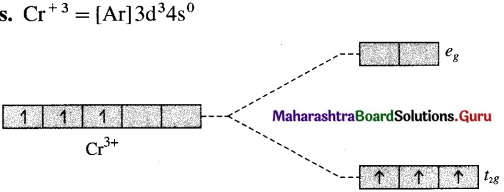Since (en) is a strong field ligand there is pairing of electrons. The electrons occupy the t2g orbitals of lower energy. It has one unpaired electron. Due to d-d transition, it is coloured.

Question i.
Give valence bond description for the hybrid orbitals are used by the metal? State the number of unpaired electrons.
Since CI is a weak ligand, there is no pairing of electrons.
Number of unpaired electrons = 2
Type of hybridisation = sp3

Geometry of complex ion = Tetrahedral
The complex ion is paramagnetic.

Question ii.
Draw a qualitatively energy-level diagram showing d-orbital splitting in the octahedral environment. Predict the number of unpaired electrons in the complex [Fe(CN)6]4⊕. Is the complex diamagnetic or paramagnetic? Is it coloured? Explain.
(A) r-orbital splitting in the octahedral environment :(B) [Fe (CN)6]4- is an octahedral complex.
(C) Since CN is a strong ligand, there is pairing of electrons and the complex is diamagnetic.
(D) The complex exists as lemon yellow crystals.
(In the complex all electrons in t2g are paired and requires high radiation energy for excitation.)

Question iii.
Draw isomers in each of the following
a. [Pt(NH3)2ClNO2]
b. [Ru(NH3)4Cl2]
c. [Cr(en2)Br2]
(a) [Pt(NH3)2CINO2]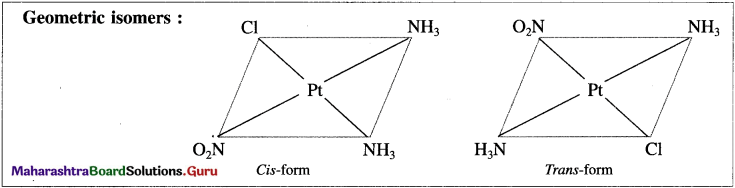(b) [RU(NH3)4CI2]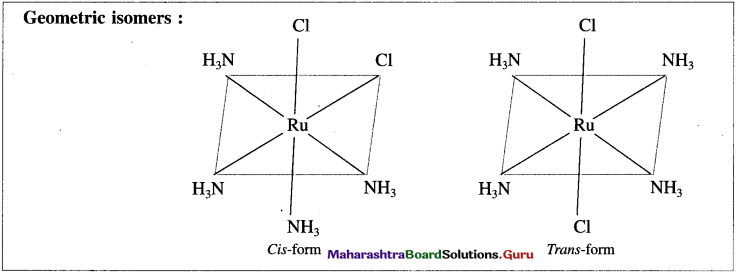(c) [Cr(en2)Br2]+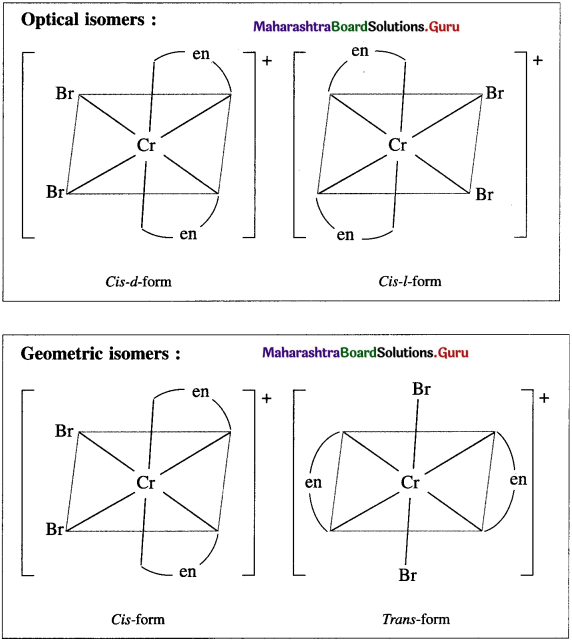Question iv.
Draw geometric isomers and enantiomers of the following complexes.
a. [Pt(en)3]4⊕
b. [Pt(en)2ClBr]2⊕
The complex [Pt(en)3]4+ has two optical isomers.Question v.
What are ligands? What are their types? Give one example of each type.
Ligands : The neutral molecules or negatively charged anions (or rarely positive ions) which are bonded by coordinate bonds to the central metal atom or metal ion in a coordination compound are called ligands or donor groups. For example in [Cu(CN)4]2-, four CN ions are ligands coordinated to central metal ion Cu2+. Ligands can be classified on the basis of number of electron donor atoms in the ligand i.e. denticity.

(1) Monodentate or unidentate ligand : A ligand molecule or an ion which has only one donor atom with a lone pair of electrons forming only one coordinate bond with metal atom or ion in the complex is called monodentate or unidentate ligand. For example NH3, Cl, OH, H2O, etc.

(2) Polydentate or multidentate ligand : A ligand molecule or an ion which has two or more donor atoms with the lone pairs of electrons forming two or more coordinate bonds with the central metal atom or ion in the complex is called polydentate or multidentate ligand. For example, ethylene diamine, H2N – (CH2)2 – NH2.
According to the number of donor atoms they are classified as follows :

• Bidentate ligand : This ligand has two donor atoms in the molecule or ion. For example, ethylenediamine, H2N – (CH2)2 – NH2.
• Tridentate ligand : This ligand molecule has three donor atoms or three sites of attachment.
E.g. Diethelene triamine, H2N – CH2 – CH2 – NH – CH2 – CH2 – NH2. This has three N donor atoms.
• Tetradentate (or quadridentate) ligand : This ligand molecule has four donor atoms.
Eg. Triethylene tetraamine which has four N donor atoms.
• Hexdentate ligand : This ligand molecule has six donor atoms. E.g. Ethylenediamine tetracetato.

(3) Ambidentate ligand : A ligand molecule or an ion which has two or more donor atoms, however in the formation of a complex, only one donor atom is attached to the metal atom or an ion is called ambidentate ligand. For example, NO2 which has two donor atoms N and O forming a coordinate bond, M ← ONO (nitrito) or M ← NO2 (nitro).

(4) Bridging ligand : A monodentate ligand having more than one lone pairs of electrons, hence can attach to two or more metal atoms or ions and hence acts as a bridge between different metal atoms is called bridging ligand. For example : OH, F, SO4-2, etc.

Question vi.
What are cationic, anionic and neutral complexes? Give one example of each.
(1) Cationic sphere complexes : A positively charged coordination sphere or a coordination compound having a positively charged coordination sphere is called cationic sphere complex.

For example : [Zn(NH3)4]2+ and [Co(NH3)5CI] SO4 are cationic complexes. The latter has coordination sphere [Co(NH3)5CI]2+, the anion SO42+ makes it electrically neutral.

(2) Anionic sphere complexes : A negatively charged coordination sphere or a coordination compound having negatively charged coordination sphere is called anionic sphere complex. For example, [Ni(CN)4]2+ and K3[Fe(CN)6] have anionic coordination sphere; [Fe(CN)6]3- and three K+ ions make the latter electrically neutral.

(3) Neutral sphere complexes : A neutral coordination complex does not possess cationic or anionic sphere.

[Pt(NH3)2CI2] or [Ni(CO)4] are neither cation nor anion but are neutral sphere complexes.Question vii.
How stability of the coordination compounds can be explained in terms of equilibrium constants?
Stability of the coordination compounds : The stability of coordination compounds can be explained on the basis of their stability constants. The stability of coordination compounds depends on metal-ligand interactions. In the complex, metal serves as electron-pair acceptor (Lewis acid) while the ligand as Lewis base (since it is electron
donor). The metal-ligand interaction can be realized as the Lewis acid-Lewis base interaction. Stronger the interaction greater is stability of the complex.

Consider the equilibrium for the metal-ligand interaction :
Ma+ + nLx- ⇌ [MLn]a+(-nx)
where a, x, [a + ( – nx)] denote the charge on the metal, ligand and the complex, respectively. Now, the equilibrium constant K is given byStability of the complex can be explained in terms of K. Higher the value of K larger is the thermodynamic stability of the complex hence K is called stability constant, and denoted by Kstah. The equilibria for the complex formation with the corresponding K values are given below.

Ag+ + 2CN ⇌ [Ag(CN)2] K = 5.5 x 1018
Cu2+ + 4CN ⇌ [CU(CN)4]2- K = 2.0 x 1027
Co3+ + 6NH3 ⇌ [CO(NH3)6]3+ K = 5.0 x 1033

From the above data, the stability of the complexes is [Co(NH3)6]3+ > [Cu(CN)4]2- > [Ag(CN)2].

Question viii.
Name the factors governing the equilibrium constants of the coordination compounds.
The equilibrium constant of the complex depends on the following factors :

(a) Charge to size ratio of the metal ion : Higher the ratio greater is the stability. For the divalent metal ion complexes their stability shows the trend : Cu2+ > Ni2+ > Co2+ > Fe2+ > Mn2+ > Cd2+. The above stability order is called the Irving-William order. In the above list both Cu and Cd have the charge + 2, however, the ionic radius of Cu2 + is 69 pm and that of Cd2 + is 97 pm. The charge to size ratio of Cu2+ is greater than that of Cd2+. Therefore the Cu2+ forms stable complexes than Cd2+.

(b) Nature of the ligand : A second factor that governs stability of the complexes is related to how easily the ligand can donate its lone pair of electrons to the central metal ion that is, the basicity of the ligand. The ligands those are stronger bases tend to form more stable complexes.

Activity :
1. The reaction of chromium metal with H 2SO4 in the absence of air gives blue solution of chromium ion.
Cr(s) + 2H(aq) → Cr2⊕(aq) + H2(s)
Cr2⊕ forms octahedral complex with H2O ligands.
a. Write formula of the complex
b. Describe bonding in the complex using CFT and VBT.
Draw crystal field splitting and valence bond orbital diagrams.

2. Reaction of complex [Co(NH3)3(NO2)3 with HCl gives a complex [Co(NH3)3H2OCl2] in which two chloride ligands are trans to one another.
a. Draw possible stereoisomers of starting material
b. Assuming that NH3 groups remain in place, which of two starting isomers would give the observed product?

12th Chemistry Digest Chapter 9 Coordination Compounds Intext Questions and Answers

Use your brain power ……. (Textbook page 192)

Question 1.
Draw Lewis structures of the following ligands and identify the donor atom in them :
NH3, H2O.Try this ………. (Textbook page 193)

Question 1.
Can you write ionisation of [Ni (NH3)6] CI2?
[Ni (NH3)6] CI2 → [Ni(NH3)6]2+ + 2CI

Question 2.
Identify coordination sphere and counter ions.
Coordination sphere : [Ni(NH3)6]2+
Counter ions : CI

Can you tell ? (Textbook page 193)

Question 1.
A complex is made of Co (III) and consists of four NH3 molecules and two CI ions as ligands. What is the charge number and formula of complexion?
The complex ion has formula, [Co(NH3)4CI2]+.
The charge number is + 1.

Use vour brain power ……………… (Textbook page 193)

Question 1.
Coordination number used in coordination of compounds is somewhat different than that used in solid state. Explain.

• In a coordination compound the coordination number is the number of donor atoms of ligands directly attached to metal atom or ion.
• In a solid state, the number of closest constituent atoms or ions in contact with a particular atom in the crystal lattice is called coordination number.
• In a coordination compound, coordination number depends upon nature of metal atom or ion, and its electronic configuration.
• In a solid state, the coordination number depends upon the crystalline structure of the unit cell.

Can you tell? ………………. (Textbook page 194)

Question 1.
What is the coordination number of
(a) Co in [CoCl2(en)2]+ = 6
(b) Ir in [Ir(C2O4)2Cl2]3+ and
(c) Pt in [Pt(NO2)2(NH3)2] ?
(a) Coordination number of Co in [CoCl2(en)2]+ = 6
(b) Coordination number of Ir in [Ir(C2O4)2Cl2]3+ = 6
(c) Coordination number of Pt in [Pt(NO2)2(NH3)2] = 4

Use your brain power ……… (Textbook page 195)

Question 1.
Classify the complexes as homoleptic and heteroleptic:
(a) [Co (NH3)5CI]SO4,
(b) [CO(ONO)(NH3)5]CI2,
(c) [CoCl(NH3)(en)2]2+ and
(d) [Cu(C2O4)3]3-
Homoleptic Complexes : (d) [Cu(C2O4)3]3-
Heteroleptic Complexes : (a) [CO(NH3)5CI]SO4
(b) [CO(ONO)(NH3)5]CI2,
(C) [CoCl(NH3)(en)2]2+

Use your brain power ……… (Textbook page 195)

Question 1.
Classify the complexes as cationic, anionic or Cr(H2O)2(C2O4)23-, PtCI2(en)2 and Cr(CO)6.
Cationic complexes : [CO(NH3)6]CI2
Anionic complexes : Na4[Fe(CN)6], [Cr(H2O)2 (C2O4)2]3-
Neutral complexes : Cr(CO)6, Pt CI2(en)2

Try this ……. (Textbook page 197)

Question 1.
Write the representation of the following :
(i) Tricarbonatocobaltate(III) ion.
(ii) Sodium hexacyanoferrate(III).
(iii) Potassium hexacyafioferrate(II).
(iv) Aquachlorobis(ethylenediamine)cobalt(III).
(vi) Diamminedichloroplatinum(II).
(i) [Co(C03)3]3-
(ii) Na3[Fe(CN)6]
(iii) K4[Fe(CN)6]
(iv) Co(en)2(H2O)(Cl)
(v) [Cr(H2O)4CI2]CI
(vi) Pt(NH3)2CI2Try this …… (Textbook page 196)

Question 1.
Find out the EAN of
(a) [Zn(NH3)4]2+
(b) [Fe(CN)6]4+
(a) For the complex ion, [Zn(NH3)4]2+ :
Atomic number of Zn = Z = 30
Charge on metal ion = + 2
∴ Number of electrons lost by Zn atom = X = 2 Total number of electrons donated by 4NH23
ligands = Y = 2 x 4 = 8
EAN = Z – X + Y
= 30 – 2 + 8
= 36

(Note : This is atomic number of the nearest inert element 36Kr.)

(b) For the complex ion, [Fe(CN)J4- :
For Fe, Z = 26 (Atomic number)
X = 2 (Due to + 2 charge on Fe)
Y = 12 (Due to 6 CN ligands)
∴ EAN = Z – X + Y
= 26 – 2 + 12
= 36

Use your brain power …… (Textbook page 197)

Question 1.
Do the following complexes follow the EAN rule
(a) Cr(CO)4,
(b) Ni(CO)4,
(c) Mn(CO)5,
(d) Fe(CO)5?
(a) Cr(CO)4 : EAN = Z – X + Y
(b) Ni(C0)4 : EAN = Z – X + Y
= 24 – 0 + 8
= 28 – 0 + 8
= 32
= 36
(c) Mn(CO)5 : EAN = Z – X + Y
= 25 – 0 + 10
= 35

(d) Fe(CO)5 : EAN = Z – X + Y
= 26 – 0 + 10
= 36

Conclusion :
(a) Cr(CO)4 and (c) Mn(CO)5 do not follow EAN Rule.Try this ….. (Textbook page 199)

Question 1.
Draw structures of ci,c and trans isomers of [Fe(NH3)2(CN)4]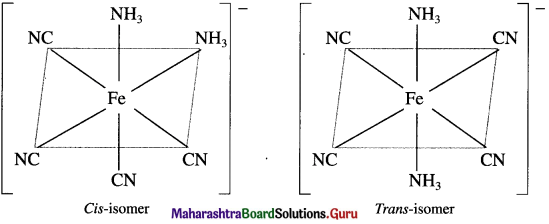Remember ….. (Textbook page 199)

Our hands are non-superimposable mirror images. When you hold your left hand up to a mirror the image looks like right hand.

Try this ….. (Textbook page 199)

Question 1.
Draw enantiomers of [Cr(OX)2]3 where OX = C2O4 :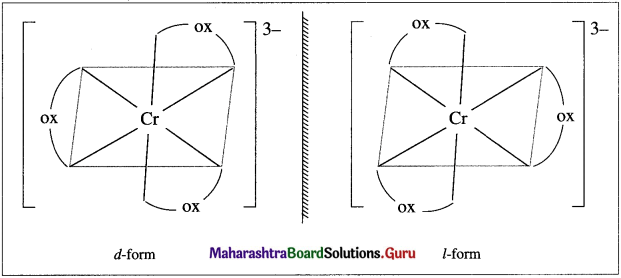Question 2.
Draw (A) enantiomers and (B) cis and trans isomers of [Cr(H2O)2(OX)2] :
(A) Enantiomers :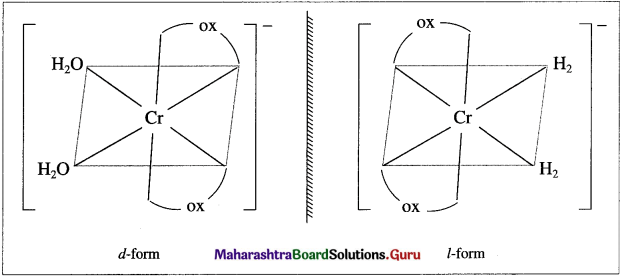(B) as and trans isomers :Can you tell ? ….. (Textbook page 200)

Question 1.
Can you write IUPAC names of isomers (I) [Co(NH3)5SO4]Br and (II) [Co(NH3)5Br]SO4?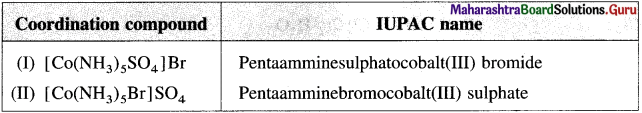Question 2.
Write linkage isomers of [Fe(H2O)5SCN]+. Write their IUPAC names.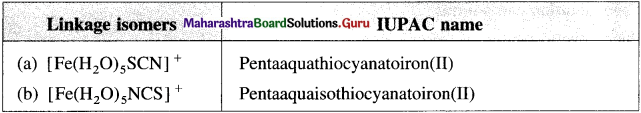Use your brain power …..(Textbook page 201)

Question 1.
The stability constant K of the [Ag(CN)2] is 5.5 x 10 while that for the corresponding [Ag(NH3)2]+ is 1.6 x 107. Explain why [Ag(CN)2]2- is more stable.
Stability constant of [Ag(CN)2]2- is larger than that of [Ag(NH3)2]+ and hence [Ag(CN)2]2- is more stable. Also, CN is a stronger ligand than NH3.

Remember …… (Textbook Page 202)

Question 1.
Complete the missing entries.(Note : The missing entries are underlined.)

Table 9.3: Type of hybridisation and geometry of a complexTry this ….. (Textbook page 204)

Question 1.
Based on the VBT predict structure and magnetic behaviour of the [Ni(NH3)6]
28Ni [Ar] 3d8 4s2
Ni3+ [Ar] 3d7 4s°
Hybridisation : sp3d2
Geometry : Octahedral
Magnetic property : ParamagneticTry this …… (Textbook page 202)

Question 1.
Give VBT description of bonding in each of following complexes. Predict their magnetic behaviour.
(a) [ZnCI4]2+
(b) [CO(H2O)6]2- (high spin)
(c) [Pt(CN)4]2- (square planar)
(d) [CoCI4]2- (tetrahedral)
(e) [Cr(NH3)6]3+

Try this ……. (Textbook page 206)

Question 1.
Sketch qualitatively crystal field d orbital energy level diagrams for each of the following complexes :
(a) [Ni(en)3]2+ (b) [Mn(CN)6]3- (c) [Fe(H2O)6]2+
Predict whether each of the complexes is diamagnetic or paramagnetic.
(a) The complex ion, [Ni(en)3]2+ is octahedral.
28Ni [Ar] 3d8 4s2
Ni2+ [Ar] 3d8 4s°.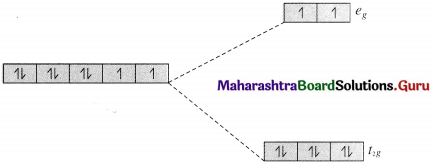Since en is a strong ligand there is pairing of electrons.
Number of unpaired electrons = n = 2 in t2g, orbitals
Magnetic moment = $$\mu=\sqrt{n(n+2)}$$
$$=\sqrt{2(2+2)}=2.83 \mathrm{~B} . \mathrm{M} .$$

The complex ion is paramagnetic.

(b) The complex ion [Mn(CN)6]3- is octahedral.
25Mn [Ar] 3d5 4s2
Mn3+ [Ar] 3d4 4s°Since CN is a strong ligand there is pairing of electrons.
Number of unpaired electrons = n = 2 in t2g, orbitals
Magnetic moment = $$\mu=\sqrt{n(n+2)}$$
$$=\sqrt{2(2+2)}=2.83 \mathrm{~B} . \mathrm{M}$$.

The complex ion is paramagnetic.

(c) The complex ion [Fe(H2O)6]2+ is octahedral.
26Fe [Ar] 3d6 4s2
Fe2+ [Ar] 3d6 45°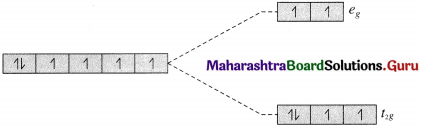Since H2O is a weak ligand, there is no pairing of electrons.
Number of unpaired electrons = n = 4 in t2g and eg orbitals.
Magnetic moment
\begin{aligned} =\mu &=\sqrt{n(n+2)} \\ &=\sqrt{4(4+2)} \\ &=4.90 \mathrm{~B} . \mathrm{M} . \end{aligned}
The complex ion is paramagnetic.